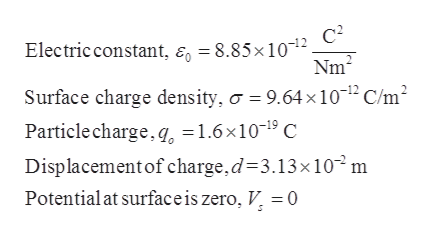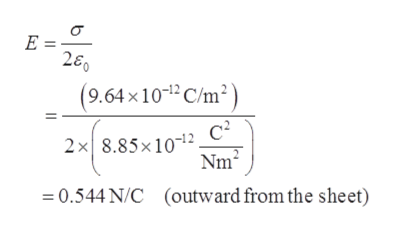# An infinite, nonconducting sheet has a surface charge density σ = +9.64 pC/m2. (a) How much work is done by the electric field due to the sheet if a particle of charge q0 = 1.60 × 10-19 C is moved from the sheet to a point P at distance d = 3.13 cm from the sheet? (b) If the electric potential V is defined to be zero on the sheet, what is V at P?

Question
619 views

An infinite, nonconducting sheet has a surface charge density σ = +9.64 pC/m2. (a) How much work is done by the electric field due to the sheet if a particle of charge q0 = 1.60 × 10-19 C is moved from the sheet to a point P at distance d = 3.13 cm from the sheet? (b) If the electric potential V is defined to be zero on the sheet, what is V at P?

check_circle

Step 1

Given,help_outlineImage TranscriptioncloseElectric constant, e = 8.85x10-2 Nm2 Surface charge density, o = 9.64 x 1012 C/m2 Particle charge, q, =1.6x1019 C Displacementof charge,d 3.13x10 m Potential at surfaceis zero, V^ = 0 fullscreen
Step 2

Electric field due to the infinite charge sheet.help_outlineImage TranscriptioncloseE - 280 (9.64x1012 C/m2 c2 2x 8.85x10-12 Nm2 (outward from the sheet) 0.544 N/C fullscreen
Step 3

Force on the charge due t...

### Want to see the full answer?

See Solution

#### Want to see this answer and more?

Solutions are written by subject experts who are available 24/7. Questions are typically answered within 1 hour.*

See Solution
*Response times may vary by subject and question.
Tagged in

### Electrostatic Potential and Capacitance